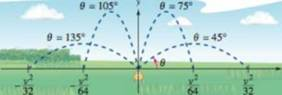Chapter 4.1, Problem 72ECalculus: Early Transcendental Fun...

7th Edition
Ron Larson + 1 other
ISBN: 9781337552516

Solutions

Chapter
SectionCalculus: Early Transcendental Fun...

7th Edition
Ron Larson + 1 other
ISBN: 9781337552516
Textbook Problem

Lawn Sprinkler A lawn sprinkler is constructed in such a way that d θ / d t is constant, where θ ranges between 45 ∘ and1 135 ∘ (see figure). The distance die water travels horizontally is x = v 2 sin 2 θ 32 , 45 ∘ ≤ θ ≤ 135 ∘ where v is the speed of the water. Find d x / d t and explain why this lawn sprinkler does not water evenly. What part of the lawn receives the most water?Water sprinkler: 45 ∘ ≤ θ ≤ 135 ∘

To determine

To calculate: The value of dxdt and explain why the lawn sprinkler does not spread water

evenly and what part of lawn receives the most water.

Explanation

Given:

The distance water travels horizontally,

x=v2sin2θ32 where 450θ1350

dθdt is constant.

And,

The figure,

Refer to figure in question.

Formula used:

AvΔT= Maximum amount of water in angle..

For maximum amount of water received: |Avdtdx|=|Avdxdt| should be largest.

Calculation:

Consider the equation,

x=v2sin2θ32

Differentiate the above equation with respect to t, to get,

dxdt=dxdθdθdt=v2cos2θ16dθdt

Lawn sprinkler does not spread the water evenly because the ratio of change of speed is not constant.

If dθdt is constant then then water will be distributed uniformly over the arc

Still sussing out bartleby?

Check out a sample textbook solution.

See a sample solution

The Solution to Your Study Problems

Bartleby provides explanations to thousands of textbook problems written by our experts, many with advanced degrees!

Get Started

Define the terms population and sample, and explain the role of each in a research study.

Essentials of Statistics for The Behavioral Sciences (MindTap Course List)

Multiply: (4)(+6)

Elementary Technical Mathematics

In Exercises 14, find the distance between the two points. (12,3) and (12,23)

Finite Mathematics for the Managerial, Life, and Social Sciences